Identical Dual Lattices and  Subdivision of Space By Ami Korren Name: Ami Korren, Architect, (b. Tiberias, Israel, 1950). Address: Faculty of Architecture & Town Planning, Technion.  Israel Institute of Technology, Technion City, Haifa 32000, Israel.  E-mail: Korren-a@inter.net.il Fields of interest:Architecture, Morphology, geometry, architectural crystallography, and Minimal and consequential forms.   Publication and exhibition:  A.Korren, Periodic 2-manifold surfaces that divide the space into two identical subspaces, Master thesis, Technion, Israel, 1993. M.Burt & A.Korren,Periodic Hyperbolic Surfaces and Subdivision of 3-Space, Katachi U Symmetry, Springer-Verlag, Tokyo, 1996. M.Burt & A.Korren, Self-Dual Space Lattices, and Periodic Hyperbolic Surfaces, Symmetry Natural and Artificial, Symmetrion Budapest, 1995. Science in the Arts Art in the Sciences, Ernst Museum, Budapest, 1999.       Introduction The morphological dealing with space subdivision by continuous 2-manifold surfaces is one of the important issues for understanding organized space and its order. The phenomenon of periodic surfaces that divide the space into two identical subspaces was dealt with over the years by various researchers from different scientific fields, such as mathematicians, architects, crystallographers, physicians, etc. These periodic 2-manifold surfaces are continuous and divide the space into two identical subspaces, which are graphically characterized by two dual tunneled space networks. These surfaces have the shape of a sponge structure. All the known surfaces were discovered in an empiric way by various scientific researchers. All in all there were seven topologically different surfaces. (Figure 1)Figure 1  The seven topologically different surfaces The goal of the research of the aforementioned phenomenon was to identify the 2-manifold, classify and exhaust them. A systematic method was suggested. The method was strongly based on the theory of symmetry groups. It was assumed that the number of surfaces, topologically different, is finite because of the fact that the number of symmetry groups is finite. Using the method for the exhaustive search the seven known topologically different surfaces were discovered. Only this time, it was done in a systematic way. Topologically different  means that the two dual tunnel networks have different shapes, which are the identification mark of the surface.  This identification of the 2-manifolds which divide the space into two identical subspaces point out that there is a direct correspondence between the continuous partition surface, the character of the two complimentary subspaces and the two self dual lattices representing them.  This phenomenon of the two identical subspaces, the surface in between, and the two self-dual lattices representing them makes the exhaustive search of any of them as one and the same problem. While studying this phenomenon the idea to search for the self-dual lattice pairs first arose for reasons that will be mentioned later. This approach led the author to discover a new unknown self-dual space network and a new 2-manifold surface in between. This new surface has the same characterization as the seven known surfaces although those seven are still unique. All the seven surfaces are the product of a simulated dipping process of a closed wire perimeter (the elementary periodic unit) in a soap solution, for producing a smooth non-self intersecting hyperbolic minimal surface. (Figure 2)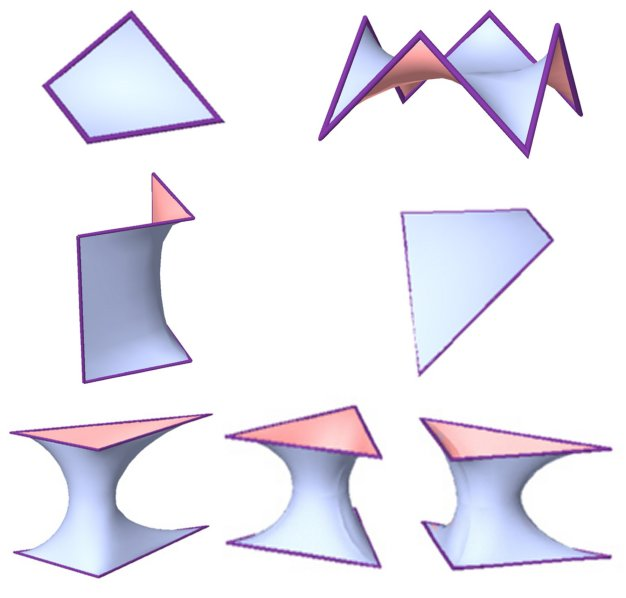Figure 2  The seven elementary periodic units. This paper intends to report on a research in which generation of dual space lattices and hyperbolic partition surfaces are at the core of the inquiry. This research is still in its initial stages. Currently, the impression is that the number of the dual and identical lattice pairs and the associated periodic hyperbolic surfaces, partitions that subdivide the entire space into two complementary and identical subspaces, is infinite.        The phenomena of 2-manifolds surfaces which  divide the space into two idenical subspaces Researching the pheonomena of periodic 2-manifolds that lie between two identical-dual subspaces led us to finding the main charasteristics of these surfaces: The surfaces are smooth and periodic and they are constructed from repetitive elemental units. The periodic 2-manifolds are created from their elemental units using symmetry. This property points out the link between the surfaces and symmetry groups. The 2-manifolds which subdivide the space into two identical subspaces represent partitions between two identical-dual lattices. The subsequent result of this congruency is the existence of symmetry operation that takes one subspace into the dual-identical one. This symmetry operation acts also on the space of the 2-manifold, which seperates between the two subspaces and is of a two-fold order. We have chosen to discuss 2-manifold with 2-fold (1800) rotation symmetry axes. These axes generate a space lattice, which should be called "the 2-fold axes lattice". This lattice is periodic, and as such has all the periodicity generating properties, meaning a 3-dimensional repetitive cell unit and elementary periodic region (E.P.R.).     The E.P.R.(Elementary Periodic Region) approach The E.P.R. is the smallest space unit (fundamental region) derived from the Euclidean space by means of the symmetry group that acts on this space. The E.P.R. contains a complete represantion of all the phenomena taking place within the "periodic complex" and particularly, representaion of the whole periodic space, its symmetry group, the 2-fold axes network, the two complementary (identical) subspaces, the self dual lattices-pair characterizing them, as well as the partition surface in between. Identification and exhaustive enumeration of the E.P.R.s are based on the fact that the number of the symmetry groups is finite (230 symmetry groups).Figure 3  Elementary Periodic Region The exhaustive method The method of exhaustive search develops in steps as follows: Exhaustive search and examination of all the possible E.P.R.s. The E.P.R. appears in 3 different shapes: Tetrahedron, Triangular prism, and Box. The vertices, edges, and faces of the E.P.R.s are the result of cutting the space by the symmetry elements.Figure 4  Typical E.P.R.s. Exhaustive search of all possible E.P.R.s capable of containing a fundametal region of 2-manifold which divide the space into two identical subspaces. A fundametal region of such 2-manifold divides the E.P.R. into two identical volumes and it includes representation of 2-fold axes which transform one subspace into the complementary dual other. It means that the E.P.R. contains a 2-fold axe(s) which rotate(s) the E.P.R. into itself. The existence of such E.P.R.s (with 2-fold axes) indicates that it is possible for those E.P.R.s to contain a fundamental region of such manifold. Repetitvely duplicating the E.P.R. (containing 2-fold axe(s)) until the resulting 2-fold axes lattice is revealed.Figure 5  Different E.P.R.s with 2-fold axesFigure 6  2-fold lattice Identifying, within the 2-fold axes lattice, the possible closed boundary perimeter(s), which could give rise to minimal surface unit (simulating the dipping operation of a wire in a soap solution). Duplication of the closed boundary perimeter with the minimal surface unit (through application of the 2-fold axes) to an extent where its general structure, its "tunnel system" and characteristic dual networks - could be identified.Figure 7  Fundamental closed boundary perimeter(s)  units with minimal surface unit(s) within.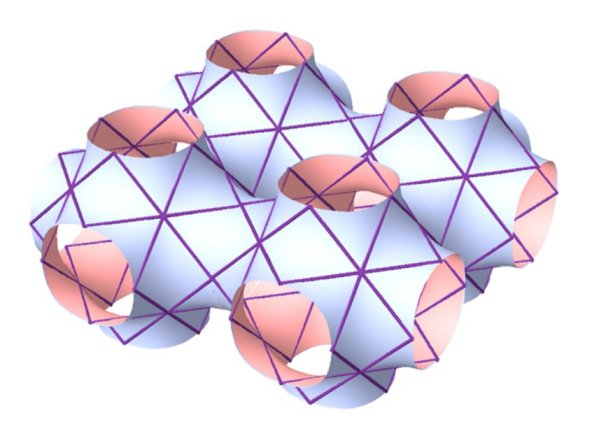Figure 8  Part of the 2-manifold, which results from a repetitive  duplication of the surface unit, which is bound by 2-fold axes. Marking the axes of the two complementary tunnels networks. Those networks are identical dual and they topologically characterize the manifold in between. Representation of the periodic 2-manifold, 2-fold axes network and the two dual lattices pair in the E.P.R.Figure 9 - Identification of the two dual networks,  determined by the periodic surface.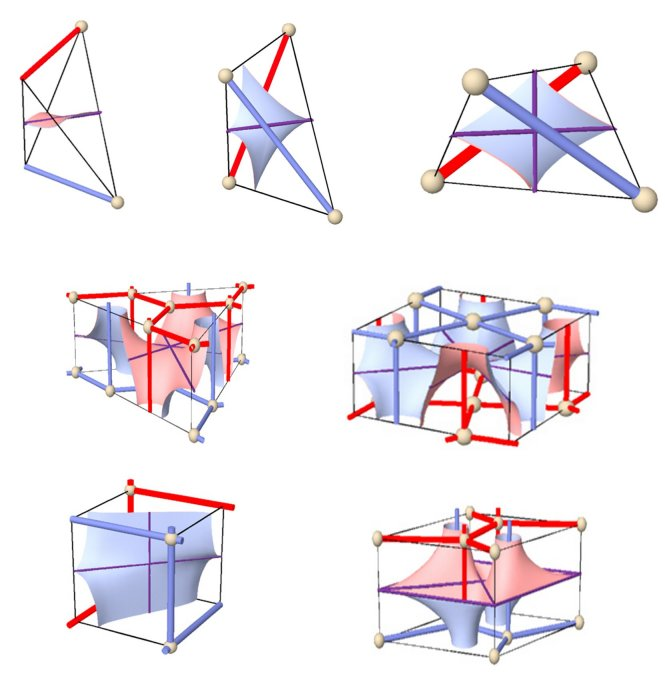Figure 10  An E.P.R. that represents all periodic elements. Classification of the 2-manifolds The aforementioned method of searching for the 2-manifolds which divide the space into two identical subspaces, lead to the discovery of many 2-manifolds, with some being topologically similar, and it also lead to exhausting all the 2-manifolds that are topologically different. There are two physical characteristics to the 2-manifolds which divide the space into two identical subspaces. One is the form of the translation cell of the 2-manifold. The other one is the geometric form of the two complementary dual subspaces, which are represented by the two dual networks.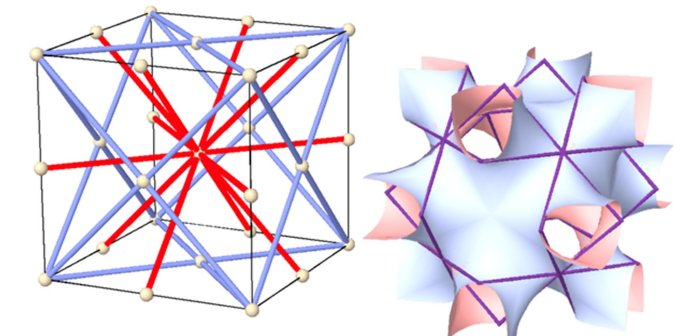Figure 11 - a. Two identical dual networks, which  characterizes the 2-manifold in (b).  b. Typical translation cell of a periodic surface that  divides between the two networks in (a). The two complementary dual subspaces have a maze-like form of two continuous tunnels. The axes of the tunnels form a continuous periodic network, which is called the "tunnel network". The relation between the two networks is complementary (dual) and each one can be defined through the other. The two aforementioned characteristics are derived from one another. The duplication of the transformation cell will lead to the creation of the 2-manifold, which divides the space to two identical subspaces, vice-versa. There is a direct, one to one correspondence between the typical translation cell and the tunnel networks. 2-manifolds, which might seem different from one another, and their fundamental region, are represented within different E.P.R.s. Similar tunnel networks may characterize them, in which case they would lead to a similar typical translation cell. 2-manifolds that are characterized by similar tunnel networks are topologically similar. Classifying the 2-manifolds according to the tunnel networks or the typical translation cell leads to discovering the 2-manifolds that are topologically different from one another. Using the aforementioned searching and classifying method lead to finding seven topologically different 2-manifolds.Figure 12  The seven two identical dual tunnel networks and the 2-manifolds that partitions them. The fact that the tunnel networks characterize the 2-manifolds on a direct, one to one correspondence leads to the conclusion that finding two self-dual networks means finding the surface partition in between. Using empiric search for dual networks we have found a new unknown pair of self-dual networks, which are topologically different from the other seven that were found by the aforementioned search method.Figure 13  New self-dual networks. The 2-manifold in between the two new self-dual networks is also topologically different from the other seven 2-manifolds. The fundamental 2-manifolds that are bounded by the 2-fold closed boundary perimeter are also unique. While the first seven 2-manifolds could be a product of a simulated dipping of a closed perimeter in a soap solution, the new one cannot.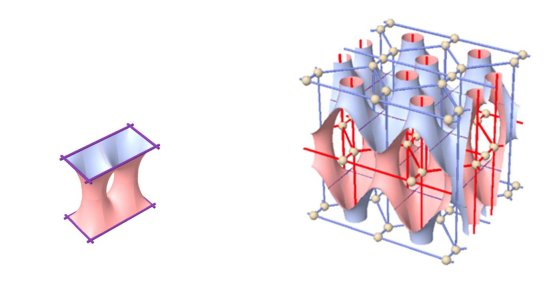Figure 14  New 2-manifold in between two self-dual networks  (right) and the fundamental 2-manifold that is bounded by  the 2-fold closed boundary perimeter (left). A new search method Finding the new self-dual network opened the door to revealing a completely new array of surface-partitions and the associated self-dual space networks, which characterize each of the two interwoven, complementary subspaces. In view of the last development, there is an advantage in shifting the gravity center of the research and pursuing the self-dual lattices pairs first. The new search method is built of several steps, and is partially based on the exhaustive method: Extracting the self-dual lattices directly from the relevant E.P.R.s (containing 2-fold rotation axes) the search of which was exhausted previously. It was done by locating the interconnected configuration of vertices and edges and its rotated image (about the 2-fold axes) within the E.P.R., in such a manner to ensure: Uninterrupted continuity of the result lattices over the whole periodic space. Duality relation between the two lattices, meaning: - 1:1 relation between edges of one of the lattices to the faces of the dual. - 1:1 relation between vertices of one of the lattices to the packing cells of the dual, and vice versa. Find the 2-manifold unit, which separates the complementary dual lattices, in a manner that assures continuity and smoothness over the boundaries of the E.P.R. and for the whole periodic hyperbolic surface. It requires that its intersection with the bounding reflection planes will be perpendicular to these planes all along the intersection curve.Figure 15  Self dual lattices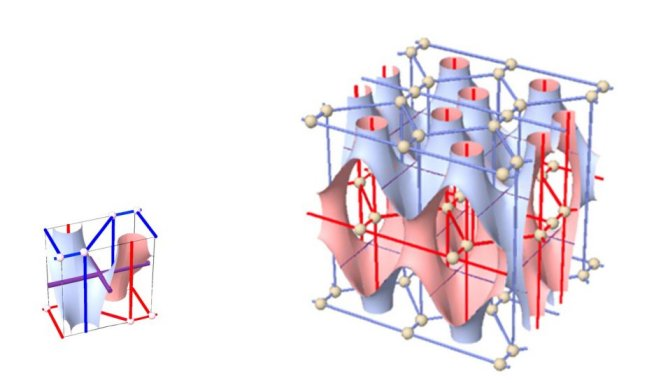Figure 16  2-manifold surface that subdivides  between the two dual networks . Conclusions Considering the apparent significance of the subject of dual lattice pairs and the subsequently related continuous Periodic Hyperbolic Surface-partitions, within the general theme of Space Subdivisions, it has never occupied a central position in a systematic and exhaustive research inquiry with these complementary spatial configurations. It should be noted that these configurations are the abstract idealized prototypes of all sponge structures, all labyrinth-like branching, infinite, enveloped, spaces and other natural phenomena, such as periodic filament configurations and continuous warped fields. They are an idealized geometric abstraction of continuous labyrinths, which shape the interiors of our modern mega-structures and our evolving urban down town environments. As of now it is clear that a general comprehensive notation system of space lattices is urgently required, as well as additional elaboration on the subject of "universal parameters" capable of capturing the nature and quality of the various ordered space lattices and related hyperbolic surfaces. It is clear also that any conspicuous advance in this research direction is conditioned by a development of a powerful and effective computerized generation and graphical representation of these lattice-surface complexes, which will be based on an input of a limited number of parameters on the simplex-E.P.R. level.     References: D.R.J. Chillingworth, Differential topology with a view to applications, Pitman Publishing London, San Francisco, Melbourne, 1976. Hilbert & Cohn-Vossen, Geometry and the imagination, Chelsea Publishing Company, New York, 1952. H.S.M. Coxeter, Regular Polytopes, Dover Publication Inc., New York, 1973 M. Burt, Spatial Arrangement and Polyhedra with Curved Surfaces and their Architectural Applications, Technion, Haifa, 1966. Peter Gay, The Crystalline State, Oliver & Boyd Edinburgh, 1972 M. Burt and A. Korren, Periodic hyperbolic surfaces and subdivision of 3-space, Hyperspace, vol. 3, #3, Japan, Dec. 1994. A. Korren, Periodic 2-manifolds surfaces, which divide the space into two identical subspaces, Master thesis, Technion, Israel, 1993.     VisMathHOME